### 6.3. Contextual boundness of rhematizers

This section contains guidelines for assigning the `tfa` values (see Section 2, "Contextual boundness") to nodes representing rhematizers. For rhematizers, the value of the attribute `tfa` is determined according to the function of the rhematizer, i.e. according to which topic-focus articulation category (topic or focus) it signals. The value of the attribute `tfa` is therefore closely related to the position of a rhematizer in the tectogrammatical tree.

A node representing a rhematizer can be assigned either the `tfa` value `t` or `f`. A rhematizer cannot be assigned the value `c`.

We suppose the following possible `tfa` values and positions for rhematizers in tectogrammatical trees:

• if the rhematizer signals the focus and the governing predicate is not in its scope (i.e. the rhematizer is placed as the closest left sister of the first node representing the expression in the scope of the rhematizer), the node representing the rhematizer is assigned the value `f`. Compare:

• Petra si umyla také.`RHEM` [`tfa`=`f`] boty [`tfa`=`f`] (=lit. Petra _ washed also (her) shoes.)

The rhematizer signals the contextually non-bound modification boty (=shoes). The node representing the rhematizer is placed as the closest left sister of the node representing the noun boty (=shoes) and is assigned the `tfa` value `f`. Cf. Fig. 10.45.

• Petra si umyla také.`RHEM` [`tfa`=`f`] červené [`tfa`=`f`] boty [`tfa`=`t`] (=lit. Petra _ washed also (her) red shoes.)

The rhematizer signals the contextually non-bound modification červený (=red) . The node representing the rhematizer is placed as the closest left sister of the node representing the adjective červený (=red) and is assigned the `tfa` value `f`. Cf. Fig. 10.46.

• if the rhematizer signals the focus and the governing predicate is in its scope (i.e. the rhematizer is placed as the closest left daughter of the node representing the predicate), the node representing the rhematizer is assigned the value `f`.

NB! In this case a contextually non-bound node (i.e. the rhematizer) is exceptionally placed to the left from its governing node (see also Section 3.1, "Basic guidelines for the ordering of nodes in a tectogrammatical tree").

Compare:

• Petra si také.`RHEM` [`tfa`=`f`] umyla [`tfa`=`f`] boty [`tfa`=`f`] (=lit. Petra _ also washed (her) shoes.)

The rhematizer signals the contextually non-bound part umyla si boty (=lit. (she) washed _ (her) shoes). The node representing the rhematizer is placed as the closest left daughter of the node representing the predicate umýt si (=to_wash) and is assigned the `tfa` value `f`. Cf. Fig. 10.47.

• if the rhematizer signals a contrastive topic (i.e. it is placed as the closest left sister of the node representing a contrastive contextually bound modification), the node representing the rhematizer is assigned the `tfa` value `t`. Compare:

• Také.`RHEM` [`tfa`=`t`] Petra [`tfa`=`c`] si umyla boty. (=lit. Also Petra _ washed (her) shoes.)

The rhematizer signals the contrastive contextually bound modification Petra. The node representing the rhematizer is placed as the closest left sister of the node representing the noun Petra and is assigned the `tfa` value `t`. Cf. Fig. 10.48.

!!! It turns out that assigning the value `t` to a node representing a rhematizer signaling a contrastive contextually bound modification (and not the value `c`) will have to be reconsidered.

• if the scope of the rhematizer comprises non-contrastive contextually bound expressions and it does not include the governing predicate (i.e. the rhematizer is placed as the closest left sister of the first node representing the expression in the scope of the rhematizer), the node representing the rhematizer is assigned the `tfa` value `t`. Compare:

• (Karel si umyl také boty. Spletl ses.) PETRA si umyla také.`RHEM` [`tfa`=`t`] boty [`tfa`=`t`] (=lit. (Charles _ washed also (his) shoes. (You) are_wrong _.) Petra _ washed (her) shoes.)

Both the rhematizer and the expression in its scope (the noun boty (=shoes) ) are non-contrastive contextually bound. The node representing the rhematizer is placed as the closest left sister of the node representing the node boty (=shoes) and is assigned the `tfa` value `t`. Cf. Fig. 10.49.

• if the scope of the rhematizer comprises a non-contrastive contextually bound governing predicate (i.e. the rhematizer is placed as the closest left daughter of the first node representing the predicate), the node representing the rhematizer is assigned the `tfa` value `t`.

This applies to constructions with a negated non-contrastive contextually bound verb (which may be e.g. repeated from the preceding context). If a negated verb is non-contrastive contextually bound, so is its negation represented as a rhematizer (see Section 6.1, "Expressions with the function of a rhematizer"). Compare:

• (Petr neodešel domů.) Hanka neodešla do školy. (=lit. (Peter did_not_go home.) Hanka did_not_go to school.)

The negated predicate is non-contrastive contextually bound. The node representing the rhematizer is assigned the `tfa` value `t`. The node for the syntactic negation (`t_lemma`=`#Neg`) is placed as the closest left daughter of the node representing the governing predicate and is likewise assigned the `tfa` value `t`.

• if the rhematizer constitutes the focus proper (see Section 3.1.1, "Focus proper"), the node representing the rhematizer is assigned the `tfa` value `f` and is placed on the rightmost path leading from the effective root of the tectogrammatical tree. All the other expressions need to be contextually bound.

• Petra si umyla boty také.`RHEM` [`tfa`=`f`] (=lit. Petra _ washed (her) shoes also.)

The rhematizer constitutes the focus proper. The node representing the rhematizer is assigned the `tfa` value `f` and is placed rightmost in the textogrammatical tree. Cf. Fig. 10.50.

Figure 10.45. Contextual boundness of rhematizers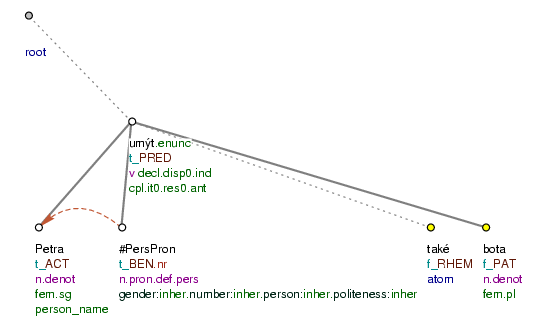Petra si umyla také boty. (=lit. Petra _ washed also (her) shoes.)

Figure 10.46. Contextual boundness of rhematizers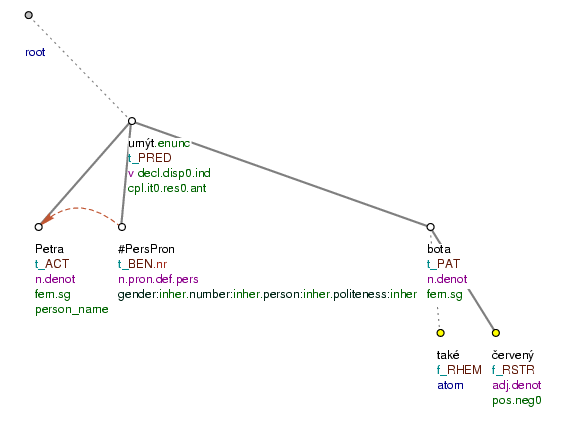Petra si umyla také červené boty. (=lit. Petra _ washed also (her) red shoes.)

Figure 10.47. Contextual boundness of rhematizers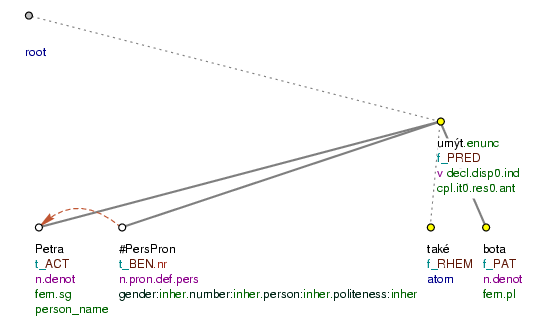Petra si také umyla boty. (=lit. Petra _ also washed (her) shoes.)

Figure 10.48. Contextual boundness of rhematizers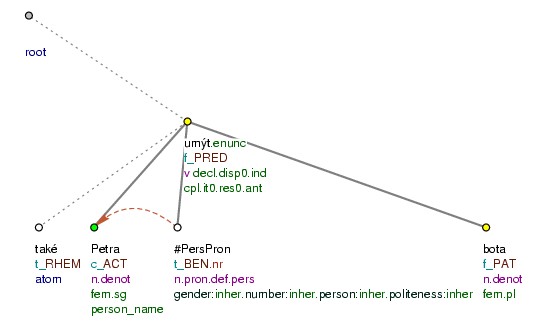Také Petra si umyla boty. (=lit. Also Petra _ washed (her) shoes.)

Figure 10.49. Contextual boundness of rhematizers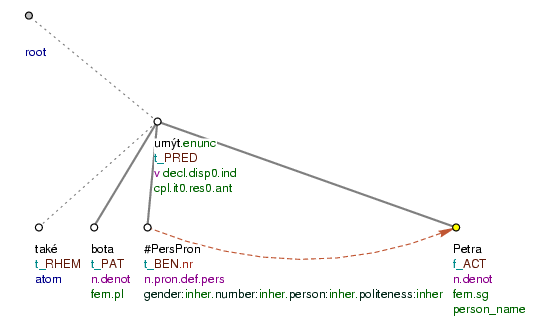(Karel si umyl také boty. Spletl ses.) Petra si umyla také boty. (=lit. (Charles _ washed also (his) shoes. (You) are_wrong _.) Petra _ washed (her) shoes.)

Figure 10.50. Contextual boundness of rhematizers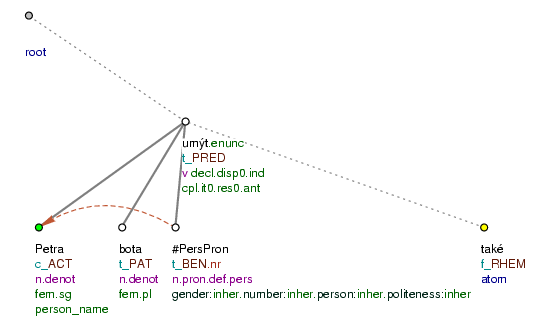Petra si umyla boty také. (=lit. Petra _ washed (her) shoes also.)